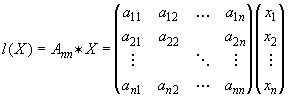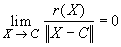#Interactive Real Analysis

Next | Previous | Glossary | Map

## 6.5. Differentiable Functions

### Examples 6.5.4(b):

Use the characterization of differentiability via approximation by linear functions to define the concept of 'derivative' for functions of n real variables.
As we have seen, we can not use the definition involving the limit of the difference quotient as a definition of 'the derivative' of a function of n real variables. Therefore, we want to try to use the 'linear approximation' characterization to give us a workable concept of derivatives in higher dimensions. First, recall that a linear function in n variables is given as matrix multiplication:
•where A is an n-by-n matrix with constant coefficients. As you recall, this is a linear map from n-dimensional space into itself. We can also define the concept of distance, or norm, in n-dimensional space as follows:

•Now we can define 'the derivative' of a function f(X) of n-variables with range in Rn:

• f: Rn--> Rn is called 'differentiable' at a point C in Rn if there exists an n-by-n matrix A such that:
• f(X) = f(C) + A * (X - C) + r(X)
• where the remainder r(X) satisfies the condition
•The matrix A is called the total derivative of the function f. It's coefficients, as you may recall from multi-dimensional calculus, are all possible partial derivatives of f at the point X = C.

We can define, in a similar fashion, the total differential of a function from n-dimensional into m-dimensional space, as long as we have the concept of a linear map and of a distance, or norm.

Next | Previous | Glossary | Map